Anzeige

# Chapter6 electronic-components

30. May 2011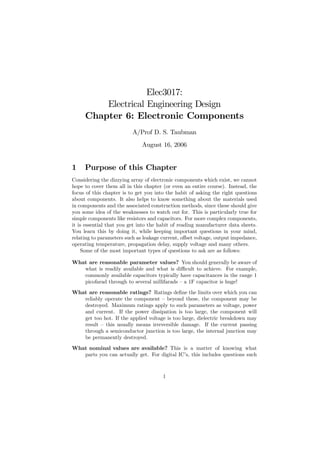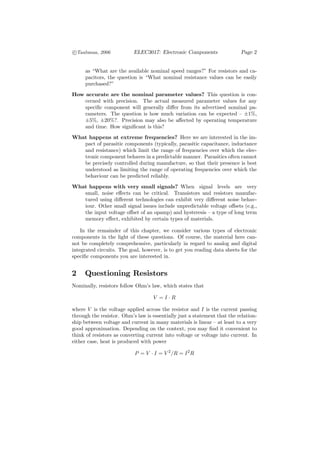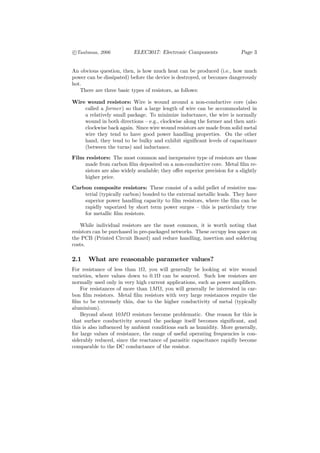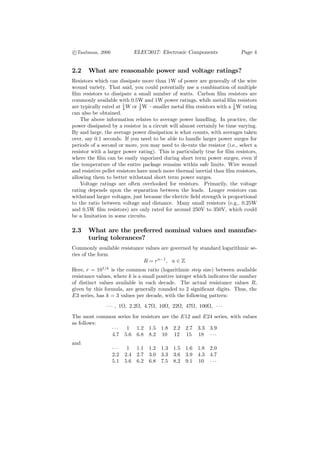Anzeige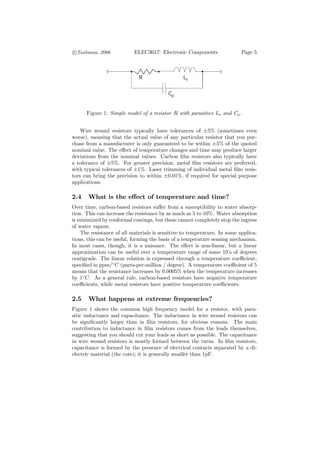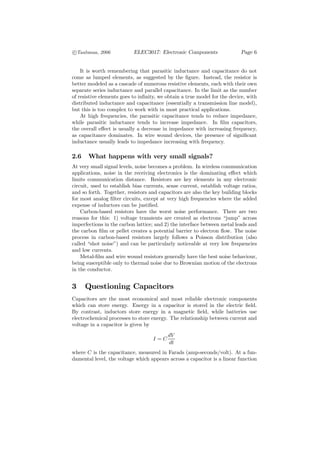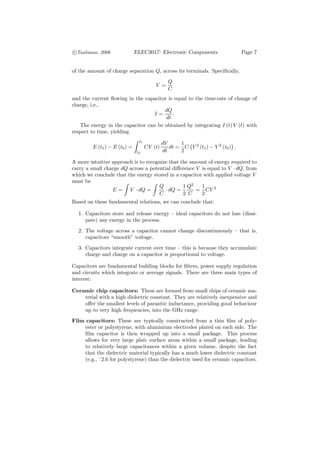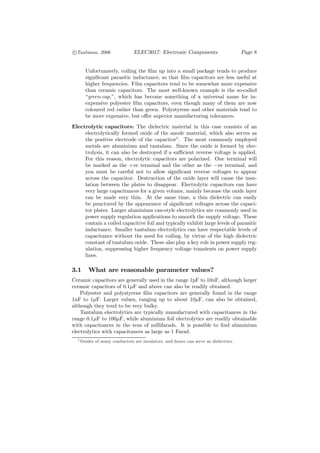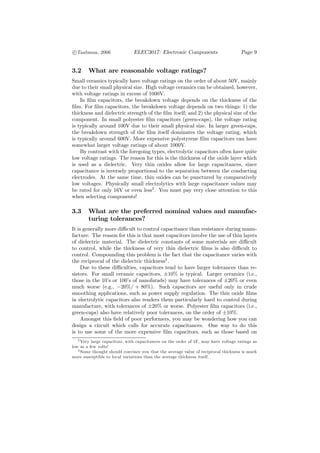Anzeige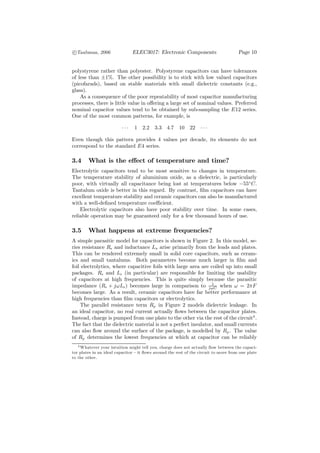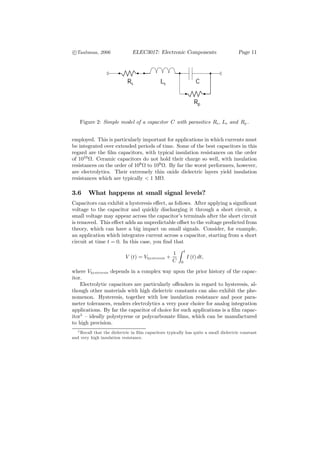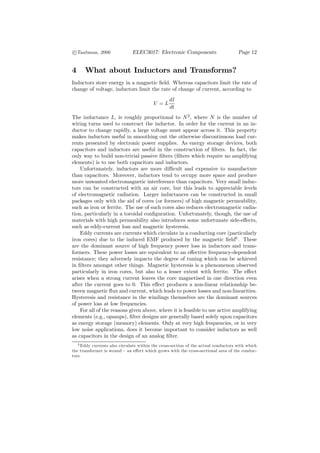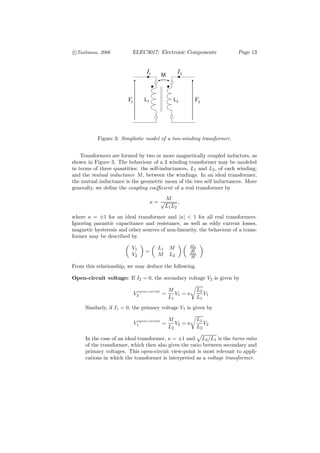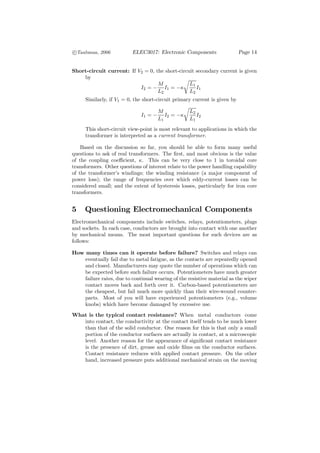Anzeige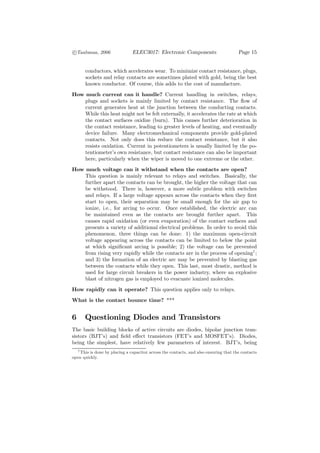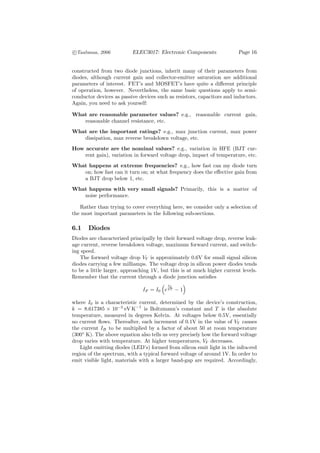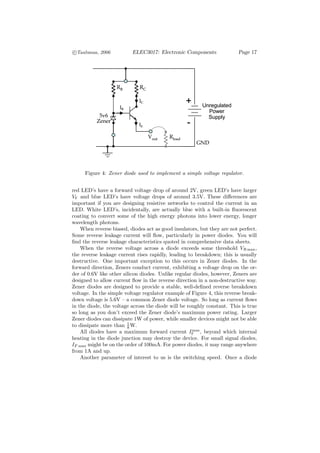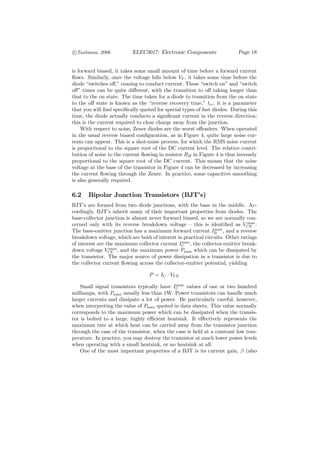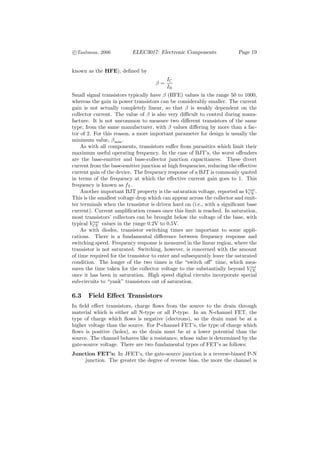Anzeige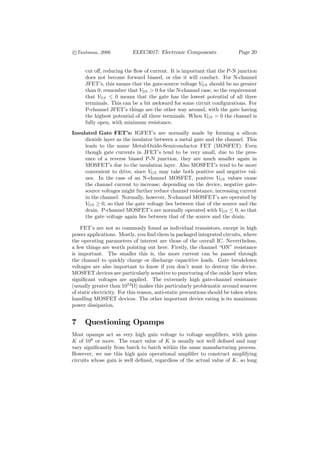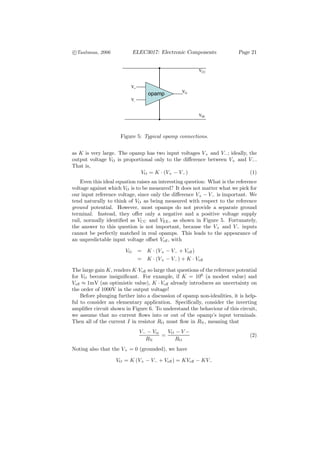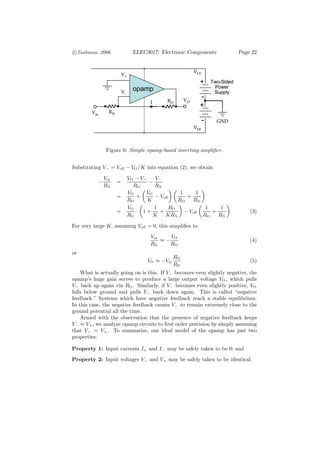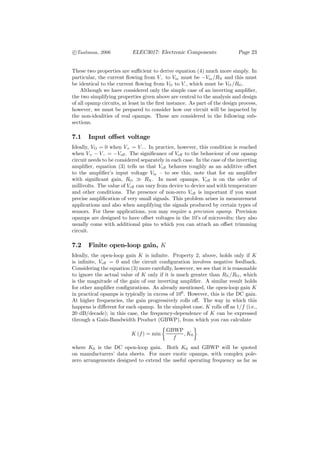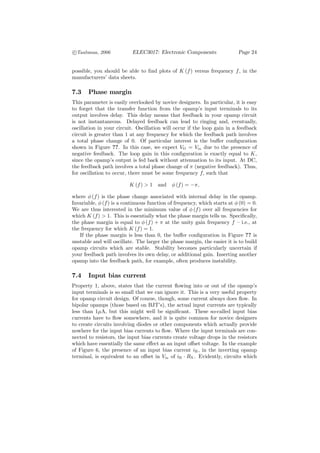Anzeige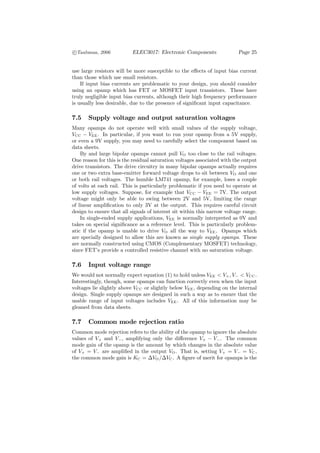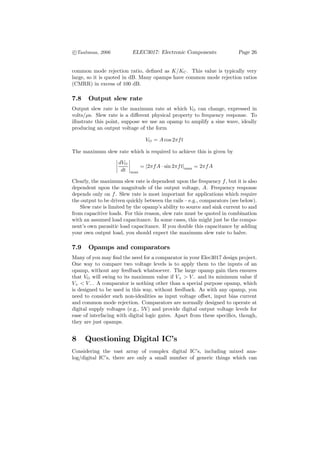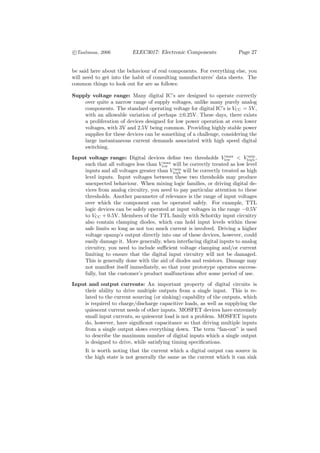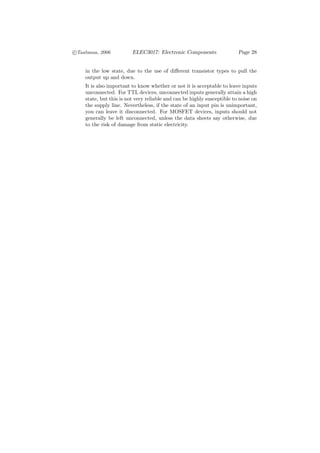Nächste SlideShare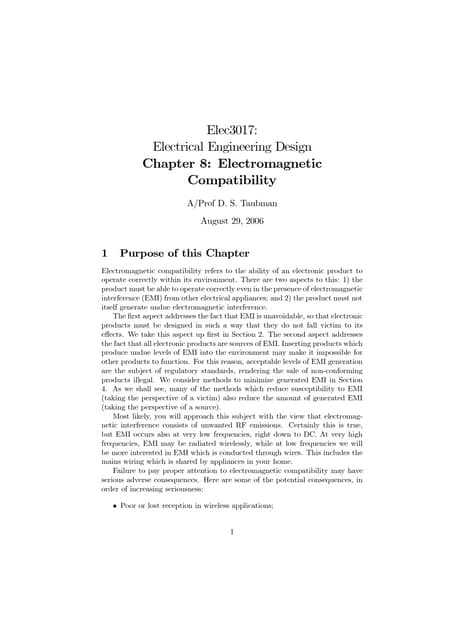Chapter8 emc
1 von 28
Anzeige

### Chapter6 electronic-components

1. Elec3017: Electrical Engineering Design Chapter 6: Electronic Components A/Prof D. S. Taubman August 16, 2006 1 Purpose of this Chapter Considering the dizzying array of electronic components which exist, we cannot hope to cover them all in this chapter (or even an entire course). Instead, the focus of this chapter is to get you into the habit of asking the right questions about components. It also helps to know something about the materials used in components and the associated construction methods, since these should give you some idea of the weaknesses to watch out for. This is particularly true for simple components like resistors and capacitors. For more complex components, it is essential that you get into the habit of reading manufacturer data sheets. You learn this by doing it, while keeping important questions in your mind, relating to parameters such as leakage current, oﬀset voltage, output impedance, operating temperature, propagation delay, supply voltage and many others. Some of the most important types of questions to ask are as follows: What are reasonable parameter values? You should generally be aware of what is readily available and what is diﬃcult to achieve. For example, commonly available capacitors typically have capacitances in the range 1 picofarad through to several millifarads — a 1F capacitor is huge! What are reasonable ratings? Ratings deﬁne the limits over which you can reliably operate the component — beyond these, the component may be destroyed. Maximum ratings apply to such parameters as voltage, power and current. If the power dissipation is too large, the component will get too hot. If the applied voltage is too large, dielectric breakdown may result — this usually means irreversible damage. If the current passing through a semiconductor junction is too large, the internal junction may be permanently destroyed. What nominal values are available? This is a matter of knowing what parts you can actually get. For digital IC’s, this includes questions such 1
2. c °Taubman, 2006 ELEC3017: Electronic Components Page 2 as “What are the available nominal speed ranges?” For resistors and ca- pacitors, the question is “What nominal resistance values can be easily purchased?” How accurate are the nominal parameter values? This question is con- cerned with precision. The actual measured parameter values for any speciﬁc component will generally diﬀer from its advertised nominal pa- rameters. The question is how much variation can be expected — ±1%, ±5%, ±20%?. Precision may also be aﬀected by operating temperature and time. How signiﬁcant is this? What happens at extreme frequencies? Here we are interested in the im- pact of parasitic components (typically, parasitic capacitance, inductance and resistance) which limit the range of frequencies over which the elec- tronic component behaves in a predictable manner. Parasitics often cannot be precisely controlled during manufacture, so that their presence is best understood as limiting the range of operating frequencies over which the behaviour can be predicted reliably. What happens with very small signals? When signal levels are very small, noise eﬀects can be critical. Transistors and resistors manufac- tured using diﬀerent technologies can exhibit very diﬀerent noise behav- iour. Other small signal issues include unpredictable voltage oﬀsets (e.g., the input voltage oﬀset of an opamp) and hysteresis — a type of long term memory eﬀect, exhibited by certain types of materials. In the remainder of this chapter, we consider various types of electronic components in the light of these questions. Of course, the material here can- not be completely comprehensive, particularly in regard to analog and digital integrated circuits. The goal, however, is to get you reading data sheets for the speciﬁc components you are interested in. 2 Questioning Resistors Nominally, resistors follow Ohm’s law, which states that V =I ·R where V is the voltage applied across the resistor and I is the current passing through the resistor. Ohm’s law is essentially just a statement that the relation- ship between voltage and current in many materials is linear — at least to a very good approximation. Depending on the context, you may ﬁnd it convenient to think of resistors as converting current into voltage or voltage into current. In either case, heat is produced with power P = V · I = V 2 /R = I 2 R
3. c °Taubman, 2006 ELEC3017: Electronic Components Page 3 An obvious question, then, is how much heat can be produced (i.e., how much power can be dissipated) before the device is destroyed, or becomes dangerously hot. There are three basic types of resistors, as follows: Wire wound resistors: Wire is wound around a non-conductive core (also called a former) so that a large length of wire can be accommodated in a relatively small package. To minimize inductance, the wire is normally wound in both directions — e.g., clockwise along the former and then anti- clockwise back again. Since wire wound resistors are made from solid metal wire they tend to have good power handling properties. On the other hand, they tend to be bulky and exhibit signiﬁcant levels of capacitance (between the turns) and inductance. Film resistors: The most common and inexpensive type of resistors are those made from carbon ﬁlm deposited on a non-conductive core. Metal ﬁlm re- sistors are also widely available; they oﬀer superior precision for a slightly higher price. Carbon composite resistors: These consist of a solid pellet of resistive ma- terial (typically carbon) bonded to the external metallic leads. They have superior power handling capacity to ﬁlm resistors, where the ﬁlm can be rapidly vaporized by short term power surges — this is particularly true for metallic ﬁlm resistors. While individual resistors are the most common, it is worth noting that resistors can be purchased in pre-packaged networks. These occupy less space on the PCB (Printed Circuit Board) and reduce handling, insertion and soldering costs. 2.1 What are reasonable parameter values? For resistance of less than 1Ω, you will generally be looking at wire wound varieties, where values down to 0.1Ω can be sourced. Such low resistors are normally used only in very high current applications, such as power ampliﬁers. For resistances of more than 1M Ω, you will generally be interested in car- bon ﬁlm resistors. Metal ﬁlm resistors with very large resistances require the ﬁlm to be extremely thin, due to the higher conductivity of metal (typically aluminium). Beyond about 10M Ω resistors become problematic. One reason for this is that surface conductivity around the package itself becomes signiﬁcant, and this is also inﬂuenced by ambient conditions such as humidity. More generally, for large values of resistance, the range of useful operating frequencies is con- siderably reduced, since the reactance of parasitic capacitance rapidly become comparable to the DC conductance of the resistor.
4. c °Taubman, 2006 ELEC3017: Electronic Components Page 4 2.2 What are reasonable power and voltage ratings? Resistors which can dissipate more than 1W of power are generally of the wire wound variety. That said, you could potentially use a combination of multiple ﬁlm resistors to dissipate a small number of watts. Carbon ﬁlm resistors are commonly available with 0.5W and 1W power ratings, while metal ﬁlm resistors are typically rated at 1 W or 1 W — smaller metal ﬁlm resistors with a 1 W rating 4 2 8 can also be obtained. The above information relates to average power handling. In practice, the power dissipated by a resistor in a circuit will almost certainly be time varying. By and large, the average power dissipation is what counts, with averages taken over, say 0.1 seconds. If you need to be able to handle larger power surges for periods of a second or more, you may need to de-rate the resistor (i.e., select a resistor with a larger power rating). This is particularly true for ﬁlm resistors, where the ﬁlm can be easily vaporized during short term power surges, even if the temperature of the entire package remains within safe limits. Wire wound and resistive pellet resistors have much more thermal inertial than ﬁlm resistors, allowing them to better withstand short term power surges. Voltage ratings are often overlooked for resistors. Primarily, the voltage rating depends upon the separation between the leads. Longer resistors can withstand larger voltages, just because the electric ﬁeld strength is proportional to the ratio between voltage and distance. Many small resistors (e.g., 0.25W and 0.5W ﬁlm resistors) are only rated for around 250V to 350V, which could be a limitation in some circuits. 2.3 What are the preferred nominal values and manufac- turing tolerances? Commonly available resistance values are governed by standard logarithmic se- ries of the form R = rn−1 , n ∈ Z Here, r = 101/k is the common ratio (logarithmic step size) between available resistance values, where k is a small positive integer which indicates the number of distinct values available in each decade. The actual resistance values R, given by this formula, are generally rounded to 2 signiﬁcant digits. Thus, the E3 series, has k = 3 values per decade, with the following pattern: · · · , 1Ω, 2.2Ω, 4.7Ω, 10Ω, 22Ω, 47Ω, 100Ω, · · · The most common series for resistors are the E12 and E24 series, with values as follows: ··· 1 1.2 1.5 1.8 2.2 2.7 3.3 3.9 4.7 5.6 6.8 8.2 10 12 15 18 · · · and ··· 1 1.1 1.2 1.3 1.5 1.6 1.8 2.0 2.2 2.4 2.7 3.0 3.3 3.6 3.9 4.3 4.7 5.1 5.6 6.2 6.8 7.5 8.2 9.1 10 · · ·
5. c °Taubman, 2006 ELEC3017: Electronic Components Page 5 R Ls Cp Figure 1: Simple model of a resistor R with parasitics Ls and Cp . Wire wound resistors typically have tolerances of ±5% (sometimes even worse), meaning that the actual value of any particular resistor that you pur- chase from a manufacturer is only guaranteed to be within ±5% of the quoted nominal value. The eﬀect of temperature changes and time may produce larger deviations from the nominal values. Carbon ﬁlm resistors also typically have a tolerance of ±5%. For greater precision, metal ﬁlm resistors are preferred, with typical tolerances of ±1%. Laser trimming of individual metal ﬁlm resis- tors can bring the precision to within ±0.01%, if required for special purpose applications. 2.4 What is the eﬀect of temperature and time? Over time, carbon-based resistors suﬀer from a susceptibility to water absorp- tion. This can increase the resistance by as much as 5 to 10%. Water absorption is minimized by conformal coatings, but these cannot completely stop the ingress of water vapour. The resistance of all materials is sensitive to temperature. In some applica- tions, this can be useful, forming the basis of a temperature sensing mechanism. In most cases, though, it is a nuisance. The eﬀect is non-linear, but a linear approximation can be useful over a temperature range of some 10’s of degrees centigrade. The linear relation is expressed through a temperature coeﬃcient, speciﬁed in ppm/◦ C (parts-per-million / degree). A temperature coeﬃcient of 5 means that the resistance increases by 0.0005% when the temperature increases by 1◦ C. As a general rule, carbon-based resistors have negative temperature coeﬃcients, while metal resistors have positive temperature coeﬃcients. 2.5 What happens at extreme frequencies? Figure 1 shows the common high frequency model for a resistor, with para- sitic inductance and capacitance. The inductance in wire wound resistors can be signiﬁcantly larger than in ﬁlm resistors, for obvious reasons. The main contribution to inductance in ﬁlm resistors comes from the leads themselves, suggesting that you should cut your leads as short as possible. The capacitance in wire wound resistors is mostly formed between the turns. In ﬁlm resistors, capacitance is formed by the presence of electrical contacts separated by a di- electric material (the core); it is generally smaller than 1pF.
6. c °Taubman, 2006 ELEC3017: Electronic Components Page 6 It is worth remembering that parasitic inductance and capacitance do not come as lumped elements, as suggested by the ﬁgure. Instead, the resistor is better modeled as a cascade of numerous resistive elements, each with their own separate series inductance and parallel capacitance. In the limit as the number of resistive elements goes to inﬁnity, we obtain a true model for the device, with distributed inductance and capacitance (essentially a transmission line model), but this is too complex to work with in most practical applications. At high frequencies, the parasitic capacitance tends to reduce impedance, while parasitic inductance tends to increase impedance. In ﬁlm capacitors, the overall eﬀect is usually a decrease in impedance with increasing frequency, as capacitance dominates. In wire wound devices, the presence of signiﬁcant inductance usually leads to impedance increasing with frequency. 2.6 What happens with very small signals? At very small signal levels, noise becomes a problem. In wireless communication applications, noise in the receiving electronics is the dominating eﬀect which limits communication distance. Resistors are key elements in any electronic circuit, used to establish bias currents, sense current, establish voltage ratios, and so forth. Together, resistors and capacitors are also the key building blocks for most analog ﬁlter circuits, except at very high frequencies where the added expense of inductors can be justiﬁed. Carbon-based resistors have the worst noise performance. There are two reasons for this: 1) voltage transients are created as electrons “jump” across imperfections in the carbon lattice; and 2) the interface between metal leads and the carbon ﬁlm or pellet creates a potential barrier to electron ﬂow. The noise process in carbon-based resistors largely follows a Poisson distribution (also called “shot noise”) and can be particularly noticeable at very low frequencies and low currents. Metal-ﬁlm and wire wound resistors generally have the best noise behaviour, being susceptible only to thermal noise due to Brownian motion of the electrons in the conductor. 3 Questioning Capacitors Capacitors are the most economical and most reliable electronic components which can store energy. Energy in a capacitor is stored in the electric ﬁeld. By contrast, inductors store energy in a magnetic ﬁeld, while batteries use electrochemical processes to store energy. The relationship between current and voltage in a capacitor is given by dV I =C dt where C is the capacitance, measured in Farads (amp-seconds/volt). At a fun- damental level, the voltage which appears across a capacitor is a linear function
7. c °Taubman, 2006 ELEC3017: Electronic Components Page 7 of the amount of charge separation Q, across its terminals. Speciﬁcally, Q V = C and the current ﬂowing in the capacitor is equal to the time-rate of change of charge, i.e., dQ I= . dt The energy in the capacitor can be obtained by integrating I (t) V (t) with respect to time, yielding Z t1 dV 1 ¡ ¢ E (t1 ) − E (t0 ) = CV (t) dt = C V 2 (t1 ) − V 2 (t0 ) . t0 dt 2 A more intuitive approach is to recognize that the amount of energy required to carry a small charge dQ across a potential diﬀerence V is equal to V · dQ, from which we conclude that the energy stored in a capacitor with applied voltage V must be Z Z Q 1 Q2 1 E = V · dQ = · dQ = = CV 2 C 2 C 2 Based on these fundamental relations, we can conclude that: 1. Capacitors store and release energy — ideal capacitors do not lose (dissi- pate) any energy in the process. 2. The voltage across a capacitor cannot change discontinuously — that is, capacitors “smooth” voltage. 3. Capacitors integrate current over time — this is because they accumulate charge and charge on a capacitor is proportional to voltage. Capacitors are fundamental building blocks for ﬁlters, power supply regulation and circuits which integrate or average signals. There are three main types of interest: Ceramic chip capacitors: These are formed from small chips of ceramic ma- terial with a high dielectric constant. They are relatively inexpensive and oﬀer the smallest levels of parasitic inductance, providing good behaviour up to very high frequencies, into the GHz range. Film capacitors: These are typically constructed from a thin ﬁlm of poly- ester or polystyrene, with aluminium electrodes plated on each side. The ﬁlm capacitor is then wrapped up into a small package. This process allows for very large plate surface areas within a small package, leading to relatively large capacitances within a given volume, despite the fact that the dielectric material typically has a much lower dielectric constant (e.g., ˜2.6 for polystyrene) than the dielectric used for ceramic capacitors.
8. c °Taubman, 2006 ELEC3017: Electronic Components Page 8 Unfortunately, coiling the ﬁlm up into a small package tends to produce signiﬁcant parasitic inductance, so that ﬁlm capacitors are less useful at higher frequencies. Film capacitors tend to be somewhat more expensive than ceramic capacitors. The most well-known example is the so-called “green-cap,”, which has become something of a universal name for in- expensive polyester ﬁlm capacitors, even though many of them are now coloured red rather than green. Polystyrene and other materials tend to be more expensive, but oﬀer superior manufacturing tolerances. Electrolytic capacitors: The dielectric material in this case consists of an electrolytically formed oxide of the anode material, which also serves as the positive electrode of the capacitor1 . The most commonly employed metals are aluminium and tantalum. Since the oxide is formed by elec- trolysis, it can also be destroyed if a suﬃcient reverse voltage is applied. For this reason, electrolytic capacitors are polarized. One terminal will be marked as the +ve terminal and the other as the −ve terminal, and you must be careful not to allow signiﬁcant reverse voltages to appear across the capacitor. Destruction of the oxide layer will cause the insu- lation between the plates to disappear. Electrolytic capacitors can have very large capacitances for a given volume, mainly because the oxide layer can be made very thin. At the same time, a thin dielectric can easily be punctured by the appearance of signiﬁcant voltages across the capaci- tor plates. Larger aluminium can-style electrolytics are commonly used in power supply regulation applications to smooth the supply voltage. These contain a coiled capacitive foil and typically exhibit large levels of parasitic inductance. Smaller tantalum electrolytics can have respectable levels of capacitance without the need for coiling, by virtue of the high dielectric constant of tantalum oxide. These also play a key role in power supply reg- ulation, suppressing higher frequency voltage transients on power supply lines. 3.1 What are reasonable parameter values? Ceramic capacitors are generally used in the range 1pF to 10nF, although larger ceramic capacitors of 0.1µF and above can also be readily obtained. Polyester and polystyrene ﬁlm capacitors are generally found in the range 1nF to 1µF. Larger values, ranging up to about 10µF, can also be obtained, although they tend to be very bulky. Tantalum electrolytics are typically manufactured with capacitances in the range 0.1µF to 100µF, while aluminium foil electrolytics are readily obtainable with capacitances in the tens of millifarads. It is possible to ﬁnd aluminium electrolytics with capacitances as large as 1 Farad. 1 Oxides of many conductors are insulators, and hence can serve as dielectrics.
9. c °Taubman, 2006 ELEC3017: Electronic Components Page 9 3.2 What are reasonable voltage ratings? Small ceramics typically have voltage ratings on the order of about 50V, mainly due to their small physical size. High voltage ceramics can be obtained, however, with voltage ratings in excess of 1000V. In ﬁlm capacitors, the breakdown voltage depends on the thickness of the ﬁlm. For ﬁlm capacitors, the breakdown voltage depends on two things: 1) the thickness and dielectric strength of the ﬁlm itself; and 2) the physical size of the component. In small polyester ﬁlm capacitors (green-caps), the voltage rating is typically around 100V due to their small physical size. In larger green-caps, the breakdown strength of the ﬁlm itself dominates the voltage rating, which is typically around 600V. More expensive polystyrene ﬁlm capacitors can have somewhat larger voltage ratings of about 1000V. By contrast with the foregoing types, electrolytic capacitors often have quite low voltage ratings. The reason for this is the thickness of the oxide layer which is used as a dielectric. Very thin oxides allow for large capacitances, since capacitance is inversely proportional to the separation between the conducting electrodes. At the same time, thin oxides can be punctured by comparatively low voltages. Physically small electrolytics with large capacitance values may be rated for only 16V or even less2 . You must pay very close attention to this when selecting components! 3.3 What are the preferred nominal values and manufac- turing tolerances? It is generally more diﬃcult to control capacitance than resistance during manu- facture. The reason for this is that most capacitors involve the use of thin layers of dielectric material. The dielectric constants of some materials are diﬃcult to control, while the thickness of very thin dielectric ﬁlms is also diﬃcult to control. Compounding this problem is the fact that the capacitance varies with the reciprocal of the dielectric thickness3 . Due to these diﬃculties, capacitors tend to have larger tolerances than re- sistors. For small ceramic capacitors, ±10% is typical. Larger ceramics (i.e., those in the 10’s or 100’s of nanofarads) may have tolerances of ±20% or even much worse (e.g., −20%/ + 80%). Such capacitors are useful only in crude smoothing applications, such as power supply regulation. The thin oxide ﬁlms in electrolytic capacitors also renders them particularly hard to control during manufacture, with tolerances of ±20% or worse. Polyester ﬁlm capacitors (i.e., green-caps) also have relatively poor tolerances, on the order of ±10%. Amongst this ﬁeld of poor performers, you may be wondering how you can design a circuit which calls for accurate capacitances. One way to do this is to use some of the more expensive ﬁlm capacitors, such as those based on 2 Very large capacitors, with capacitances on the order of 1F, may have voltage ratings as low as a few volts! 3 Some thought should convince you that the average value of reciprocal thickness is much more susceptible to local variations than the average thickness itself.
10. c °Taubman, 2006 ELEC3017: Electronic Components Page 10 polystyrene rather than polyester. Polystyrene capacitors can have tolerances of less than ±1%. The other possibility is to stick with low valued capacitors (picofarads), based on stable materials with small dielectric constants (e.g., glass). As a consequence of the poor repeatability of most capacitor manufacturing processes, there is little value in oﬀering a large set of nominal values. Preferred nominal capacitor values tend to be obtained by sub-sampling the E12 series. One of the most common patterns, for example, is ··· 1 2.2 3.3 4.7 10 22 · · · Even though this pattern provides 4 values per decade, its elements do not correspond to the standard E4 series. 3.4 What is the eﬀect of temperature and time? Electrolytic capacitors tend to be most sensitive to changes in temperature. The temperature stability of aluminium oxide, as a dielectric, is particularly poor, with virtually all capacitance being lost at temperatures below −55◦ C. Tantalum oxide is better in this regard. By contrast, ﬁlm capacitors can have excellent temperature stability and ceramic capacitors can also be manufactured with a well-deﬁned temperature coeﬃcient. Electrolytic capacitors also have poor stability over time. In some cases, reliable operation may be guaranteed only for a few thousand hours of use. 3.5 What happens at extreme frequencies? A simple parasitic model for capacitors is shown in Figure 2. In this model, se- ries resistance Rs and inductance Ls arise primarily from the leads and plates. This can be rendered extremely small in solid core capacitors, such as ceram- ics and small tantalums. Both parameters become much larger in ﬁlm and foil electrolytics, where capacitive foils with large area are coiled up into small packages. Rs and Ls (in particular) are responsible for limiting the usability of capacitors at high frequencies. This is quite simply because the parasitic 1 impedance (Rs + jωLs ) becomes large in comparison to jωC when ω = 2πF becomes large. As a result, ceramic capacitors have far better performance at high frequencies than ﬁlm capacitors or electrolytics. The parallel resistance term Rp in Figure 2 models dielectric leakage. In an ideal capacitor, no real current actually ﬂows between the capacitor plates. Instead, charge is pumped from one plate to the other via the rest of the circuit4 . The fact that the dielectric material is not a perfect insulator, and small currents can also ﬂow around the surface of the package, is modelled by Rp . The value of Rp determines the lowest frequencies at which at capacitor can be reliably 4 Whatever your intuition might tell you, charge does not actually ﬂow between the capaci- tor plates in an ideal capacitor — it ﬂows around the rest of the circuit to move from one plate to the other.
11. c °Taubman, 2006 ELEC3017: Electronic Components Page 11 Rs Ls C Rp Figure 2: Simple model of a capacitor C with parasitics Rs , Ls and Rp . employed. This is particularly important for applications in which currents must be integrated over extended periods of time. Some of the best capacitors in this regard are the ﬁlm capacitors, with typical insulation resistances on the order of 1010 Ω. Ceramic capacitors do not hold their charge so well, with insulation resistances on the order of 108 Ω to 109 Ω. By far the worst performers, however, are electrolytics. Their extremely thin oxide dielectric layers yield insulation resistances which are typically < 1 MΩ. 3.6 What happens at small signal levels? Capacitors can exhibit a hysteresis eﬀect, as follows. After applying a signiﬁcant voltage to the capacitor and quickly discharging it through a short circuit, a small voltage may appear across the capacitor’s terminals after the short circuit is removed. This eﬀect adds an unpredictable oﬀset to the voltage predicted from theory, which can have a big impact on small signals. Consider, for example, an application which integrates current across a capacitor, starting from a short circuit at time t = 0. In this case, you ﬁnd that Z 1 t V (t) = Vhysteresis + I (t) dt, C 0 where Vhysteresis depends in a complex way upon the prior history of the capac- itor. Electrolytic capacitors are particularly oﬀenders in regard to hysteresis, al- though other materials with high dielectric constants can also exhibit the phe- nomenon. Hysteresis, together with low insulation resistance and poor para- meter tolerances, renders electrolytics a very poor choice for analog integration applications. By far the capacitor of choice for such applications is a ﬁlm capac- itor5 — ideally polystyrene or polycarbonate ﬁlms, which can be manufactured to high precision. 5 Recall that the dielectric in ﬁlm capacitors typically has quite a small dielectric constant and very high insulation resistance.
12. c °Taubman, 2006 ELEC3017: Electronic Components Page 12 4 What about Inductors and Transforms? Inductors store energy in a magnetic ﬁeld. Whereas capacitors limit the rate of change of voltage, inductors limit the rate of change of current, according to dI V =L dt The inductance L, is roughly proportional to N 2 , where N is the number of wiring turns used to construct the inductor. In order for the current in an in- ductor to change rapidly, a large voltage must appear across it. This property makes inductors useful in smoothing out the otherwise discontinuous load cur- rents presented by electronic power supplies. As energy storage devices, both capacitors and inductors are useful in the construction of ﬁlters. In fact, the only way to build non-trivial passive ﬁlters (ﬁlters which require no amplifying elements) is to use both capacitors and inductors. Unfortunately, inductors are more diﬃcult and expensive to manufacture than capacitors. Moreover, inductors tend to occupy more space and produce more unwanted electromagnetic interference than capacitors. Very small induc- tors can be constructed with an air core, but this leads to appreciable levels of electromagnetic radiation. Larger inductances can be constructed in small packages only with the aid of cores (or formers) of high magnetic permeability, such as iron or ferrite. The use of such cores also reduces electromagnetic radia- tion, particularly in a toroidal conﬁguration. Unfortunately, though, the use of materials with high permeability also introduces some unfortunate side-eﬀects, such as eddy-current loss and magnetic hysteresis. Eddy currents are currents which circulate in a conducting core (particularly iron cores) due to the induced EMF produced by the magnetic ﬁeld6 . These are the dominant source of high frequency power loss in inductors and trans- formers. These power losses are equivalent to an eﬀective frequency-dependent resistance; they adversely impacts the degree of tuning which can be achieved in ﬁlters amongst other things. Magnetic hysteresis is a phenomenon observed particularly in iron cores, but also to a lesser extent with ferrite. The eﬀect arises when a strong current leaves the core magnetised in one direction even after the current goes to 0. This eﬀect produces a non-linear relationship be- tween magnetic ﬂux and current, which leads to power losses and non-linearities. Hysteresis and resistance in the windings themselves are the dominant sources of power loss at low frequencies. For all of the reasons given above, where it is feasible to use active amplifying elements (e.g., opamps), ﬁlter designs are generally based solely upon capacitors as energy storage (memory) elements. Only at very high frequencies, or in very low noise applications, does it become important to consider inductors as well as capacitors in the design of an analog ﬁlter. 6 Eddy currents also circulate within the cross-section of the actual conductors with which the transformer is wound — an eﬀect which grows with the cross-sectional area of the conduc- tors.
13. c °Taubman, 2006 ELEC3017: Electronic Components Page 13 I1 I2 M V1 L1 L2 V2 Figure 3: Simplistic model of a two-winding transformer. Transformers are formed by two or more magnetically coupled inductors, as shown in Figure 3. The behaviour of a 2 winding transformer may be modeled in terms of three quantities: the self-inductances, L1 and L2 , of each winding; and the mutual inductance M , between the windings. In an ideal transformer, the mutual inductance is the geometric mean of the two self inductances. More generally, we deﬁne the coupling coeﬃcient of a real transformer by M κ= √ , L1 L2 where κ = ±1 for an ideal transformer and |κ| < 1 for all real transformers. Ignoring parasitic capacitance and resistance, as well as eddy current losses, magnetic hysteresis and other sources of non-linearity, the behaviour of a trans- former may be described by µ ¶ µ ¶ µ dI1 ¶ V1 L1 M dt = dI2 V2 M L2 dt From this relationship, we may deduce the following. Open-circuit voltage: If I2 = 0, the secondary voltage V2 is given by r op en-circuit M L2 V2 = V1 = κ V1 L1 L1 Similarly, if I1 = 0, the primary voltage V1 is given by r M L1 V1op en-circuit = V2 = κ V2 L2 L2 p In the case of an ideal transformer, κ = ±1 and L2 /L1 is the turns ratio of the transformer, which then also gives the ratio between secondary and primary voltages. This open-circuit view-point is most relevant to appli- cations in which the transformer is interpreted as a voltage transformer.
14. c °Taubman, 2006 ELEC3017: Electronic Components Page 14 Short-circuit current: If V2 = 0, the short-circuit secondary current is given by r M L1 I2 = − I1 = −κ I1 L2 L2 Similarly, if V1 = 0, the short-circuit primary current is given by r M L2 I1 = − I2 = −κ I2 L1 L1 This short-circuit view-point is most relevant to applications in which the transformer is interpreted as a current transformer. Based on the discussion so far, you should be able to form many useful questions to ask of real transformers. The ﬁrst, and most obvious is the value of the coupling coeﬃcient, κ. This can be very close to 1 in toroidal core transformers. Other questions of interest relate to the power handling capability of the transformer’s windings; the winding resistance (a major component of power loss); the range of frequencies over which eddy-current losses can be considered small; and the extent of hysteresis losses, particularly for iron core transformers. 5 Questioning Electromechanical Components Electromechanical components include switches, relays, potentiometers, plugs and sockets. In each case, conductors are brought into contact with one another by mechanical means. The most important questions for such devices are as follows: How many times can it operate before failure? Switches and relays can eventually fail due to metal fatigue, as the contacts are repeatedly opened and closed. Manufacturers may quote the number of operations which can be expected before such failure occurs. Potentiometers have much greater failure rates, due to continual wearing of the resistive material as the wiper contact moves back and forth over it. Carbon-based potentiometers are the cheapest, but fail much more quickly than their wire-wound counter- parts. Most of you will have experienced potentiometers (e.g., volume knobs) which have become damaged by excessive use. What is the typical contact resistance? When metal conductors come into contact, the conductivity at the contact itself tends to be much lower than that of the solid conductor. One reason for this is that only a small portion of the conductor surfaces are actually in contact, at a microscopic level. Another reason for the appearance of signiﬁcant contact resistance is the presence of dirt, grease and oxide ﬁlms on the conductor surfaces. Contact resistance reduces with applied contact pressure. On the other hand, increased pressure puts additional mechanical strain on the moving
15. c °Taubman, 2006 ELEC3017: Electronic Components Page 15 conductors, which accelerates wear. To minimize contact resistance, plugs, sockets and relay contacts are sometimes plated with gold, being the best known conductor. Of course, this adds to the cost of manufacture. How much current can it handle? Current handling in switches, relays, plugs and sockets is mainly limited by contact resistance. The ﬂow of current generates heat at the junction between the conducting contacts. While this heat might not be felt externally, it accelerates the rate at which the contact surfaces oxidize (burn). This causes further deterioration in the contact resistance, leading to greater levels of heating, and eventually device failure. Many electromechanical components provide gold-plated contacts. Not only does this reduce the contact resistance, but it also resists oxidation. Current in potentiometers is usually limited by the po- tentiometer’s own resistance, but contact resistance can also be important here, particularly when the wiper is moved to one extreme or the other. How much voltage can it withstand when the contacts are open? This question is mainly relevant to relays and switches. Basically, the further apart the contacts can be brought, the higher the voltage that can be withstood. There is, however, a more subtle problem with switches and relays. If a large voltage appears across the contacts when they ﬁrst start to open, their separation may be small enough for the air gap to ionize, i.e., for arcing to occur. Once established, the electric arc can be maintained even as the contacts are brought further apart. This causes rapid oxidation (or even evaporation) of the contact surfaces and presents a variety of additional electrical problems. In order to avoid this phenomenon, three things can be done: 1) the maximum open-circuit voltage appearing across the contacts can be limited to below the point at which signiﬁcant arcing is possible; 2) the voltage can be prevented from rising very rapidly while the contacts are in the process of opening7 ; and 3) the formation of an electric arc may be prevented by blasting gas between the contacts while they open. This last, most drastic, method is used for large circuit breakers in the power industry, where an explosive blast of nitrogen gas is employed to evacuate ionized molecules. How rapidly can it operate? This question applies only to relays. What is the contact bounce time? *** 6 Questioning Diodes and Transistors The basic building blocks of active circuits are diodes, bipolar junction tran- sistors (BJT’s) and ﬁeld eﬀect transistors (FET’s and MOSFET’s). Diodes, being the simplest, have relatively few parameters of interest. BJT’s, being 7 This is done by placing a capacitor across the contacts, and also ensuring that the contacts open quickly.
16. c °Taubman, 2006 ELEC3017: Electronic Components Page 16 constructed from two diode junctions, inherit many of their parameters from diodes, although current gain and collector-emitter saturation are additional parameters of interest. FET’s and MOSFET’s have quite a diﬀerent principle of operation, however. Nevertheless, the same basic questions apply to semi- conductor devices as passive devices such as resistors, capacitors and inductors. Again, you need to ask yourself: What are reasonable parameter values? e.g., reasonable current gain, reasonable channel resistance, etc. What are the important ratings? e.g., max junction current, max power dissipation, max reverse breakdown voltage, etc. How accurate are the nominal values? e.g., variation in HFE (BJT cur- rent gain), variation in forward voltage drop, impact of temperature, etc. What happens at extreme frequencies? e.g., how fast can my diode turn on; how fast can it turn on; at what frequency does the eﬀective gain from a BJT drop below 1, etc. What happens with very small signals? Primarily, this is a matter of noise performance. Rather than trying to cover everything here, we consider only a selection of the most important parameters in the following sub-sections. 6.1 Diodes Diodes are characterized principally by their forward voltage drop, reverse leak- age current, reverse breakdown voltage, maximum forward current, and switch- ing speed. The forward voltage drop VF is approximately 0.6V for small signal silicon diodes carrying a few milliamps. The voltage drop in silicon power diodes tends to be a little larger, approaching 1V, but this is at much higher current levels. Remember that the current through a diode junction satisﬁes ³ VF ´ IF = I0 e kT − 1 where I0 is a characteristic current, determined by the device’s construction, k = 8.617385 × 10−5 eV K−1 is Boltzmann’s constant and T is the absolute temperature, measured in degrees Kelvin. At voltages below 0.5V, essentially no current ﬂows. Thereafter, each increment of 0.1V in the value of VF causes the current IB to be multiplied by a factor of about 50 at room temperature (300◦ K). The above equation also tells us very precisely how the forward voltage drop varies with temperature. At higher temperatures, VF decreases. Light emitting diodes (LED’s) formed from silicon emit light in the infra-red region of the spectrum, with a typical forward voltage of around 1V. In order to emit visible light, materials with a larger band-gap are required. Accordingly,
17. c °Taubman, 2006 ELEC3017: Electronic Components Page 17 RB RC IC + Unregulated IB Power 5v6 Supply Zener - IE Vout Rload GND Figure 4: Zener diode used to implement a simple voltage regulator. red LED’s have a forward voltage drop of around 2V, green LED’s have larger VF and blue LED’s have voltage drops of around 3.5V. These diﬀerences are important if you are designing resistive networks to control the current in an LED. White LED’s, incidentally, are actually blue with a built-in ﬂuorescent coating to convert some of the high energy photons into lower energy, longer wavelength photons. When reverse biased, diodes act as good insulators, but they are not perfect. Some reverse leakage current will ﬂow, particularly in power diodes. You will ﬁnd the reverse leakage characteristics quoted in comprehensive data sheets. When the reverse voltage across a diode exceeds some threshold VR max , the reverse leakage current rises rapidly, leading to breakdown; this is usually destructive. One important exception to this occurs in Zener diodes. In the forward direction, Zeners conduct current, exhibiting a voltage drop on the or- der of 0.6V like other silicon diodes. Unlike regular diodes, however, Zeners are designed to allow current ﬂow in the reverse direction in a non-destructive way. Zener diodes are designed to provide a stable, well-deﬁned reverse breakdown voltage. In the simple voltage regulator example of Figure 4, this reverse break- down voltage is 5.6V — a common Zener diode voltage. So long as current ﬂows in the diode, the voltage across the diode will be roughly constant. This is true so long as you don’t exceed the Zener diode’s maximum power rating. Larger Zener diodes can dissipate 1W of power, while smaller devices might not be able to dissipate more than 1 W. 8 max All diodes have a maximum forward current IF , beyond which internal heating in the diode junction may destroy the device. For small signal diodes, IF max might be on the order of 100mA. For power diodes, it may range anywhere from 1A and up. Another parameter of interest to us is the switching speed. Once a diode
18. c °Taubman, 2006 ELEC3017: Electronic Components Page 18 is forward biased, it takes some small amount of time before a forward current ﬂows. Similarly, once the voltage falls below VF , it takes some time before the diode “switches oﬀ,” ceasing to conduct current. These “switch on” and “switch oﬀ” times can be quite diﬀerent, with the transition to oﬀ taking longer than that to the on state. The time taken for a diode to transition from the on state to the oﬀ state is known as the “reverse recovery time,” trr ; it is a parameter that you will ﬁnd speciﬁcally quoted for special types of fast diodes. During this time, the diode actually conducts a signiﬁcant current in the reverse direction; this is the current required to clear charge away from the junction. With respect to noise, Zener diodes are the worst oﬀenders. When operated in the usual reverse biased conﬁguration, as in Figure 4, quite large noise cur- rents can appear. This is a shot-noise process, for which the RMS noise current is proportional to the square root of the DC current level. The relative contri- bution of noise to the current ﬂowing in resistor RB in Figure 4 is thus inversely proportional to the square root of the DC current. This means that the noise voltage at the base of the transistor in Figure 4 can be decreased by increasing the current ﬂowing through the Zener. In practice, some capacitive smoothing is also generally required. 6.2 Bipolar Junction Transistors (BJT’s) BJT’s are formed from two diode junctions, with the base in the middle. Ac- cordingly, BJT’s inherit many of their important properties from diodes. The base-collector junction is almost never forward biased, so we are normally con- max cerned only with its reverse breakdown voltage — this is identiﬁed as VCB . max The base-emitter junction has a maximum forward current IB , and a reverse breakdown voltage, which are both of interest in practical circuits. Other ratings max of interest are the maximum collector current IC , the collector-emitter break- max down voltage VCE , and the maximum power Pmax which can be dissipated by the transistor. The major source of power dissipation in a transistor is due to the collector current ﬂowing across the collector-emitter potential, yielding P = IC · VCE max Small signal transistors typically have IC values of one or two hundred milliamps, with Pmax usually less than 1W. Power transistors can handle much larger currents and dissipate a lot of power. Be particularly careful, however, when interpreting the value of Pmax quoted in data sheets. This value normally corresponds to the maximum power which can be dissipated when the transis- tor is bolted to a large, highly eﬃcient heatsink. It eﬀectively represents the maximum rate at which heat can be carried away from the transistor junction through the case of the transistor, when the case is held at a constant low tem- perature. In practice, you may destroy the transistor at much lower power levels when operating with a small heatsink, or no heatsink at all. One of the most important properties of a BJT is its current gain, β (also
19. c °Taubman, 2006 ELEC3017: Electronic Components Page 19 known as the HFE), deﬁned by IC β= IB Small signal transistors typically have β (HFE) values in the range 50 to 1000, whereas the gain in power transistors can be considerably smaller. The current gain is not actually completely linear, so that β is weakly dependent on the collector current. The value of β is also very diﬃcult to control during manu- facture. It is not uncommon to measure two diﬀerent transistors of the same type, from the same manufacturer, with β values diﬀering by more than a fac- tor of 2. For this reason, a more important parameter for design is usually the minimum value, β min . As with all components, transistors suﬀer from parasitics which limit their maximum useful operating frequency. In the case of BJT’s, the worst oﬀenders are the base-emitter and base-collector junction capacitances. These divert current from the base-emitter junction at high frequencies, reducing the eﬀective current gain of the device. The frequency response of a BJT is commonly quoted in terms of the frequency at which the eﬀective current gain goes to 1. This frequency is known as fT . sat Another important BJT property is the saturation voltage, reported as VCE . This is the smallest voltage drop which can appear across the collector and emit- ter terminals when the transistor is driven hard on (i.e., with a signiﬁcant base current). Current ampliﬁcation ceases once this limit is reached. In saturation, most transistors’ collectors can be brought below the voltage of the base, with sat typical VCE values in the range 0.2V to 0.5V. As with diodes, transistor switching times are important to some appli- cations. There is a fundamental diﬀerence between frequency response and switching speed. Frequency response is measured in the linear region, where the transistor is not saturated. Switching, however, is concerned with the amount of time required for the transistor to enter and subsequently leave the saturated condition. The longer of the two times is the “switch oﬀ” time, which mea- sat sures the time taken for the collector voltage to rise substantially beyond VCE once it has been in saturation. High speed digital circuits incorporate special sub-circuits to “yank” transistors out of saturation. 6.3 Field Eﬀect Transistors In ﬁeld eﬀect transistors, charge ﬂows from the source to the drain through material which is either all N-type or all P-type. In an N-channel FET, the type of charge which ﬂows is negative (electrons), so the drain must be at a higher voltage than the source. For P-channel FET’s, the type of charge which ﬂows is positive (holes), so the drain must be at a lower potential than the source. The channel behaves like a resistance, whose value is determined by the gate-source voltage. There are two fundamental types of FET’s as follows: Junction FET’s: In JFET’s, the gate-source junction is a reverse-biased P-N junction. The greater the degree of reverse bias, the more the channel is
20. c °Taubman, 2006 ELEC3017: Electronic Components Page 20 cut oﬀ, reducing the ﬂow of current. It is important that the P-N junction does not become forward biased, or else it will conduct. For N-channel JFET’s, this means that the gate-source voltage VGS should be no greater than 0; remember that VDS > 0 for the N-channel case, so the requirement that VGS ≤ 0 means that the gate has the lowest potential of all three terminals. This can be a bit awkward for some circuit conﬁgurations. For P-channel JFET’s things are the other way around, with the gate having the highest potential of all three terminals. When VGS = 0 the channel is fully open, with minimum resistance. Insulated Gate FET’s: IGFET’s are normally made by forming a silicon dioxide layer as the insulator between a metal gate and the channel. This leads to the name Metal-Oxide-Semiconductor FET (MOSFET). Even though gate currents in JFET’s tend to be very small, due to the pres- ence of a reverse biased P-N junction, they are much smaller again in MOSFET’s due to the insulation layer. Also MOSFET’s tend to be more convenient to drive, since VGS may take both positive and negative val- ues. In the case of an N-channel MOSFET, positive VGS values cause the channel current to increase; depending on the device, negative gate- source voltages might further reduce channel resistance, increasing current in the channel. Normally, however, N-channel MOSFET’s are operated by VGS ≥ 0, so that the gate voltage lies between that of the source and the drain. P-channel MOSFET’s are normally operated with VGS ≤ 0, so that the gate voltage again lies between that of the source and the drain. FET’s are not so commonly found as individual transistors, except in high power applications. Mostly, you ﬁnd them in packaged integrated circuits, where the operating parameters of interest are those of the overall IC. Nevertheless, a few things are worth pointing out here. Firstly, the channel “ON” resistance is important. The smaller this is, the more current can be passed through the channel to quickly charge or discharge capacitive loads. Gate breakdown voltages are also important to know if you don’t want to destroy the device. MOSFET devices are particularly sensitive to puncturing of the oxide layer when signiﬁcant voltages are applied. The extremely high gate-channel resistance (usually greater than 1012 Ω) makes this particularly problematic around sources of static electricity. For this reason, anti-static precautions should be taken when handling MOSFET devices. The other important device rating is its maximum power dissipation. 7 Questioning Opamps Most opamps act as very high gain voltage to voltage ampliﬁers, with gains K of 106 or more. The exact value of K is usually not well deﬁned and may vary signiﬁcantly from batch to batch within the same manufacturing process. However, we use this high gain operational ampliﬁer to construct amplifying circuits whose gain is well deﬁned, regardless of the actual value of K, so long
21. c °Taubman, 2006 ELEC3017: Electronic Components Page 21 VCC V+ VO opamp V- VEE Figure 5: Typical opamp connections. as K is very large. The opamp has two input voltages V+ and V− ; ideally, the output voltage VO is proportional only to the diﬀerence between V+ and V− . That is, VO = K · (V+ − V− ) (1) Even this ideal equation raises an interesting question: What is the reference voltage against which VO is to be measured? It does not matter what we pick for our input reference voltage, since only the diﬀerence V+ − V− is important. We tend naturally to think of VO as being measured with respect to the reference ground potential. However, most opamps do not provide a separate ground terminal. Instead, they oﬀer only a negative and a positive voltage supply rail, normally identiﬁed as VCC and VEE , as shown in Figure 5. Fortunately, the answer to this question is not important, because the V+ and V− inputs cannot be perfectly matched in real opamps. This leads to the appearance of an unpredictable input voltage oﬀset Voﬀ , with VO = K · (V+ − V− + Voﬀ ) = K · (V+ − V− ) + K · Voﬀ The large gain K, renders K·Voﬀ so large that questions of the reference potential for VO become insigniﬁcant. For example, if K = 106 (a modest value) and Voﬀ ≈ 1mV (an optimistic value), K · Voﬀ already introduces an uncertainty on the order of 1000V in the output voltage! Before plunging further into a discussion of opamp non-idealities, it is help- ful to consider an elementary application. Speciﬁcally, consider the inverting ampliﬁer circuit shown in Figure 6. To understand the behaviour of this circuit, we assume that no current ﬂows into or out of the opamp’s input terminals. Then all of the current I in resistor RO must ﬂow in RN , meaning that V− − Vin VO − V − = (2) RN RO Noting also that the V+ = 0 (grounded), we have VO = K (V+ − V− + Voﬀ ) = KVoﬀ − KV−
22. c °Taubman, 2006 ELEC3017: Electronic Components Page 22 VCC V+ + Two-Sided opamp Power V- Supply RO VO - I + Vin RN - GND VEE Figure 6: Simple opamp-based inverting ampliﬁer. Substituting V− = Voﬀ − VO /K into equation (2), we obtain Vin VO − V− V− − = − RN RO RN µ ¶µ ¶ VO VO 1 1 = + − Voﬀ + RO K RO RN µ ¶ µ ¶ VO 1 RO 1 1 = · 1+ + − Voﬀ + (3) RO K KRN RO RN For very large K, assuming Voﬀ = 0, this simpliﬁes to Vin VO ≈− (4) RN RO or RO VO ≈ −Vin (5) RN What is actually going on is this. If V− becomes even slightly negative, the opamp’s huge gain serves to produce a large output voltage VO , which pulls V− back up again via RO . Similarly, if V− becomes even slightly positive, VO falls below ground and pulls V− back down again. This is called “negative feedback.” Systems which have negative feedback reach a stable equilibrium. In this case, the negative feedback causes V− to remain extremely close to the ground potential all the time. Armed with the observation that the presence of negative feedback keeps V− ≈ V+ , we analyze opamp circuits to ﬁrst order precision by simply assuming that V− = V+ . To summarize, our ideal model of the opamp has just two properties: Property 1: Input currents I+ and I− may be safely taken to be 0; and Property 2: Input voltages V− and V+ may be safely taken to be identical.
23. c °Taubman, 2006 ELEC3017: Electronic Components Page 23 These two properties are suﬃcient to derive equation (4) much more simply. In particular, the current ﬂowing from V− to Vin must be −Vin /RN and this must be identical to the current ﬂowing from VO to V- , which must be VO /RO . Although we have considered only the simple case of an inverting ampliﬁer, the two simplifying properties given above are central to the analysis and design of all opamp circuits, at least in the ﬁrst instance. As part of the design process, however, we must be prepared to consider how our circuit will be impacted by the non-idealities of real opamps. These are considered in the following sub- sections. 7.1 Input oﬀset voltage Ideally, VO = 0 when V+ = V− . In practice, however, this condition is reached when V+ − V− = −Voﬀ . The signiﬁcance of Voﬀ to the behaviour of our opamp circuit needs to be considered separately in each case. In the case of the inverting ampliﬁer, equation (3) tells us that Voﬀ behaves roughly as an additive oﬀset to the ampliﬁer’s input voltage Vin — to see this, note that for an ampliﬁer with signiﬁcant gain, RO À RN . In most opamps, Voﬀ is on the order of millivolts. The value of Voﬀ can vary from device to device and with temperature and other conditions. The presence of non-zero Voﬀ is important if you want precise ampliﬁcation of very small signals. This problem arises in measurement applications and also when amplifying the signals produced by certain types of sensors. For these applications, you may require a precision opamp. Precision opamps are designed to have oﬀset voltages in the 10’s of microvolts; they also usually come with additional pins to which you can attach an oﬀset trimming circuit. 7.2 Finite open-loop gain, K Ideally, the open-loop gain K is inﬁnite. Property 2, above, holds only if K is inﬁnite, Voﬀ = 0 and the circuit conﬁguration involves negative feedback. Considering the equation (3) more carefully, however, we see that it is reasonable to ignore the actual value of K only if it is much greater than RN /RO , which is the magnitude of the gain of our inverting ampliﬁer. A similar result holds for other ampliﬁer conﬁgurations. As already mentioned, the open-loop gain K in practical opamps is typically in excess of 106 . However, this is the DC gain. At higher frequencies, the gain progressively rolls oﬀ. The way in which this happens is diﬀerent for each opamp. In the simplest case, K rolls oﬀ as 1/f (i.e., 20 dB/decade); in this case, the frequency-dependence of K can be expressed through a Gain-Bandwidth Product (GBWP), from which you can calculate ½ ¾ GBWP K (f ) = min , K0 f where K0 is the DC open-loop gain. Both K0 and GBWP will be quoted on manufacturers’ data sheets. For more exotic opamps, with complex pole- zero arrangements designed to extend the useful operating frequency as far as
24. c °Taubman, 2006 ELEC3017: Electronic Components Page 24 possible, you should be able to ﬁnd plots of K (f ) versus frequency f , in the manufacturers’ data sheets. 7.3 Phase margin This parameter is easily overlooked by novice designers. In particular, it is easy to forget that the transfer function from the opamp’s input terminals to its output involves delay. This delay means that feedback in your opamp circuit is not instantaneous. Delayed feedback can lead to ringing and, eventually, oscillation in your circuit. Oscillation will occur if the loop gain in a feedback circuit is greater than 1 at any frequency for which the feedback path involves a total phase change of 0. Of particular interest is the buﬀer conﬁguration shown in Figure ??. In this case, we expect VO = Vin due to the presence of negative feedback. The loop gain in this conﬁguration is exactly equal to K, since the opamp’s output is fed back without attenuation to its input. At DC, the feedback path involves a total phase change of π (negative feedback). Thus, for oscillation to occur, there must be some frequency f, such that K (f ) > 1 and φ (f ) = −π, where φ (f ) is the phase change associated with internal delay in the opamp. Invariable, φ (f ) is a continuous function of frequency, which starts at φ (0) = 0. We are thus interested in the minimum value of φ (f ) over all frequencies for which K (f ) > 1. This is essentially what the phase margin tells us. Speciﬁcally, the phase margin is equal to φ (f ) + π at the unity gain frequency f — i.e., at the frequency for which K (f ) = 1. If the phase margin is less than 0, the buﬀer conﬁguration in Figure ?? is unstable and will oscillate. The larger the phase margin, the easier it is to build opamp circuits which are stable. Stability becomes particularly uncertain if your feedback path involves its own delay, or additional gain. Inserting another opamp into the feedback path, for example, often produces instability. 7.4 Input bias current Property 1, above, states that the current ﬂowing into or out of the opamp’s input terminals is so small that we can ignore it. This is a very useful property for opamp circuit design. Of course, though, some current always does ﬂow. In bipolar opamps (those based on BJT’s), the actual input currents are typically less than 1µA, but this might well be signiﬁcant. These so-called input bias currents have to ﬂow somewhere, and it is quite common for novice designers to create circuits involving diodes or other components which actually provide nowhere for the input bias currents to ﬂow. Where the input terminals are con- nected to resistors, the input bias currents create voltage drops in the resistors which have essentially the same eﬀect as an input oﬀset voltage. In the example of Figure 6, the presence of an input bias current iB , in the inverting opamp terminal, is equivalent to an oﬀset in Vin of iB · RN . Evidently, circuits which
25. c °Taubman, 2006 ELEC3017: Electronic Components Page 25 use large resistors will be more susceptible to the eﬀects of input bias current than those which use small resistors. If input bias currents are problematic to your design, you should consider using an opamp which has FET or MOSFET input transistors. These have truly negligible input bias currents, although their high frequency performance is usually less desirable, due to the presence of signiﬁcant input capacitance. 7.5 Supply voltage and output saturation voltages Many opamps do not operate well with small values of the supply voltage, VCC − VEE . In particular, if you want to run your opamp from a 5V supply, or even a 9V supply, you may need to carefully select the component based on data sheets. By and large bipolar opamps cannot pull VO too close to the rail voltages. One reason for this is the residual saturation voltages associated with the output drive transistors. The drive circuitry in many bipolar opamps actually requires one or two extra base-emitter forward voltage drops to sit between VO and one or both rail voltages. The humble LM741 opamp, for example, loses a couple of volts at each rail. This is particularly problematic if you need to operate at low supply voltages. Suppose, for example that VCC − VEE = 7V. The output voltage might only be able to swing between 2V and 5V, limiting the range of linear ampliﬁcation to only 3V at the output. This requires careful circuit design to ensure that all signals of interest sit within this narrow voltage range. In single-ended supply applications, VEE is normally interpreted as 0V and takes on special signiﬁcance as a reference level. This is particularly problem- atic if the opamp is unable to drive VO all the way to VEE . Opamps which are specially designed to allow this are known as single supply opamps. These are normally constructed using CMOS (Complementary MOSFET) technology, since FET’s provide a controlled resistive channel with no saturation voltage. 7.6 Input voltage range We would not normally expect equation (1) to hold unless VEE < V+ , V− < VCC . Interestingly, though, some opamps can function correctly even when the input voltages lie slightly above VCC or slightly below VEE , depending on the internal design. Single supply opamps are designed in such a way as to ensure that the usable range of input voltages includes VEE . All of this information may be gleaned from data sheets. 7.7 Common mode rejection ratio Common mode rejection refers to the ability of the opamp to ignore the absolute values of V+ and V− , amplifying only the diﬀerence V+ − V− . The common mode gain of the opamp is the amount by which changes in the absolute value of V+ = V− are ampliﬁed in the output VO . That is, setting V+ = V− = VC , the common mode gain is KC = ∆VO /∆VC . A ﬁgure of merit for opamps is the
26. c °Taubman, 2006 ELEC3017: Electronic Components Page 26 common mode rejection ratio, deﬁned as K/KC . This value is typically very large, so it is quoted in dB. Many opamps have common mode rejection ratios (CMRR) in excess of 100 dB. 7.8 Output slew rate Output slew rate is the maximum rate at which VO can change, expressed in volts/µs. Slew rate is a diﬀerent physical property to frequency response. To illustrate this point, suppose we use an opamp to amplify a sine wave, ideally producing an output voltage of the form VO = A cos 2πf t The maximum slew rate which is required to achieve this is given by ¯ ¯ ¯ dVO ¯ ¯ ¯ = |2πf A · sin 2πf t|max = 2πf A ¯ dt ¯ max Clearly, the maximum slew rate is dependent upon the frequency f , but it is also dependent upon the magnitude of the output voltage, A. Frequency response depends only on f . Slew rate is most important for applications which require the output to be driven quickly between the rails — e.g., comparators (see below). Slew rate is limited by the opamp’s ability to source and sink current to and from capacitive loads. For this reason, slew rate must be quoted in combination with an assumed load capacitance. In some cases, this might just be the compo- nent’s own parasitic load capacitance. If you double this capacitance by adding your own output load, you should expect the maximum slew rate to halve. 7.9 Opamps and comparators Many of you may ﬁnd the need for a comparator in your Elec3017 design project. One way to compare two voltage levels is to apply them to the inputs of an opamp, without any feedback whatsoever. The large opamp gain then ensures that VO will swing to its maximum value if V+ > V− and its minimum value if V+ < V− . A comparator is nothing other than a special purpose opamp, which is designed to be used in this way, without feedback. As with any opamp, you need to consider such non-idealities as input voltage oﬀset, input bias current and common mode rejection. Comparators are normally designed to operate at digital supply voltages (e.g., 5V) and provide digital output voltage levels for ease of interfacing with digital logic gates. Apart from these speciﬁcs, though, they are just opamps. 8 Questioning Digital IC’s Considering the vast array of complex digital IC’s, including mixed ana- log/digital IC’s, there are only a small number of generic things which can
27. c °Taubman, 2006 ELEC3017: Electronic Components Page 27 be said here about the behaviour of real components. For everything else, you will need to get into the habit of consulting manufacturers’ data sheets. The common things to look out for are as follows: Supply voltage range: Many digital IC’s are designed to operate correctly over quite a narrow range of supply voltages, unlike many purely analog components. The standard operating voltage for digital IC’s is VCC = 5V, with an allowable variation of perhaps ±0.25V. These days, there exists a proliferation of devices designed for low power operation at even lower voltages, with 3V and 2.5V being common. Providing highly stable power supplies for these devices can be something of a challenge, considering the large instantaneous current demands associated with high speed digital switching. max min Input voltage range: Digital devices deﬁne two thresholds Vlow < Vhigh , max such that all voltages less than Vlow will be correctly treated as low level min inputs and all voltages greater than Vhigh will be correctly treated as high level inputs. Input voltages between these two thresholds may produce unexpected behaviour. When mixing logic families, or driving digital de- vices from analog circuitry, you need to pay particular attention to these thresholds. Another parameter of relevance is the range of input voltages over which the component can be operated safely. For example, TTL logic devices can be safely operated at input voltages in the range −0.5V to VCC + 0.5V. Members of the TTL family with Schottky input circuitry also contain clamping diodes, which can hold input levels within these safe limits so long as not too much current is involved. Driving a higher voltage opamp’s output directly into one of these devices, however, could easily damage it. More generally, when interfacing digital inputs to analog circuitry, you need to include suﬃcient voltage clamping and/or current limiting to ensure that the digital input circuitry will not be damaged. This is generally done with the aid of diodes and resistors. Damage may not manifest itself immediately, so that your prototype operates success- fully, but the customer’s product malfunctions after some period of use. Input and output currents: An important property of digital circuits is their ability to drive multiple outputs from a single input. This is re- lated to the current sourcing (or sinking) capability of the outputs, which is required to charge/discharge capacitive loads, as well as supplying the quiescent current needs of other inputs. MOSFET devices have extremely small input currents, so quiescent load is not a problem. MOSFET inputs do, however, have signiﬁcant capacitance so that driving multiple inputs from a single output slows everything down. The term “fan-out” is used to describe the maximum number of digital inputs which a single output is designed to drive, while satisfying timing speciﬁcations. It is worth noting that the current which a digital output can source in the high state is not generally the same as the current which it can sink
28. c °Taubman, 2006 ELEC3017: Electronic Components Page 28 in the low state, due to the use of diﬀerent transistor types to pull the output up and down. It is also important to know whether or not it is acceptable to leave inputs unconnected. For TTL devices, unconnected inputs generally attain a high state, but this is not very reliable and can be highly susceptible to noise on the supply line. Nevertheless, if the state of an input pin is unimportant, you can leave it disconnected. For MOSFET devices, inputs should not generally be left unconnected, unless the data sheets say otherwise, due to the risk of damage from static electricity.
Anzeige